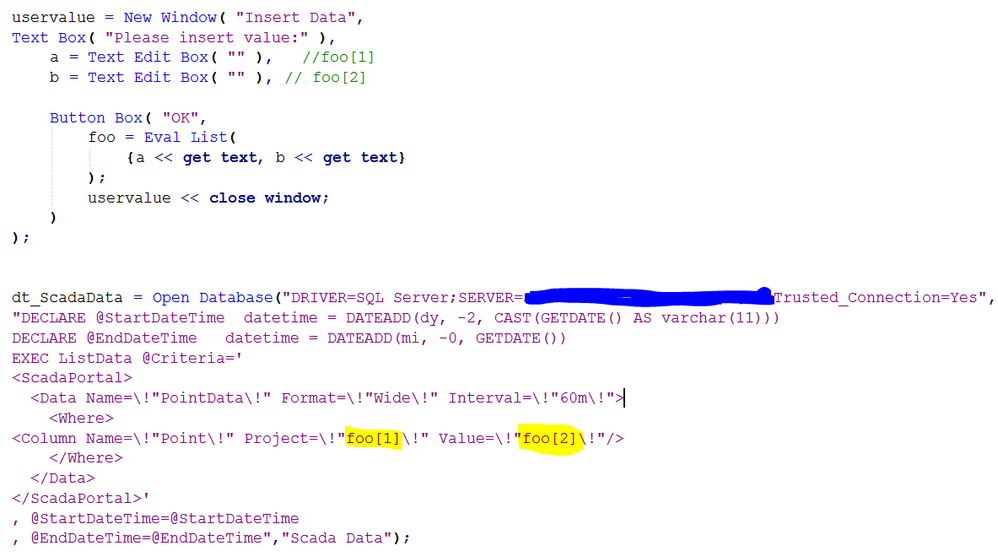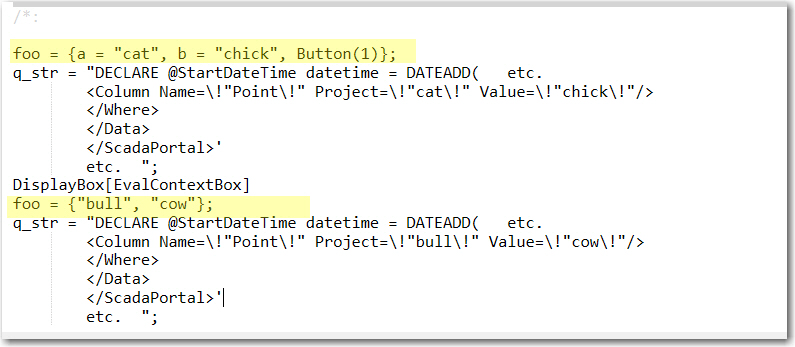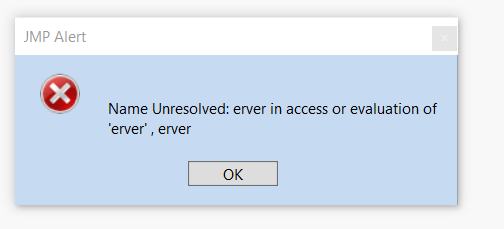Our World Statistics Day conversations have been a great reminder of how much statistics can inform our lives. Do you have an example of how statistics has made a difference in your life? Share your story with the Community!
Choose Language Hide Translation Bar
Highlighted

## A variable value from user - sql query

I try to get a variable value from the user and use it as a SQL query
How can I add to a query?

Thanks!1 ACCEPTED SOLUTION

Accepted Solutions
Highlighted

## Re: A variable value from user - sql query

@Botzal, please search for Open Database() in the JMP Scripting Index >> Functions. This function has 2 required and 2 option arguments:

1. the connect string
2. the query string
3.  <invisible | private>  property for the output table, you likely do not want this
4. <output table name>

You have been using one string for the first two arguments.  It is difficult to debug and see this when we do not have test files to use. Remove the comment lines for dt_ScadaData to test if it runs. Remove the comment lines for NW if you want the UI to automatically close. You might want it to remain open so you can pull several tables. If that is what you want, then Eval Insert Into(qstr),  replaces the place holder variables foo and foo.   So just in case you intend to use the UI more than once, I created a variable cq_str which is assigned the variable string. So this should work.  I typically do not use Eval Insert Into() but instead something like cq_str = Eval Insert(q_str);

``````LB = Lineup Box(1);
LB << Append(
PB_Inputs = Panel Box("User Inputs",
a = Text Edit Box( "" ), //foo
b = Text Edit Box( "" ), // foo
);
);
q_connect = "DRIVER=SQL Server;SERVER=MyServer;Trusted_Connection=Yes";
q_str = "DECLARE @StartDateTime  datetime = DATEADD(day, -2, CAST(GETDATE() AS varchar(11)))
DECLARE @EndDateTime   datetime = DATEADD(day,0, GETDATE())
EXEC ListData @Criteria=
<Data Name=\!"PointData\!" Format=\!"Wide\!" Interval=\!"60m\!">
<Where>
<Column Name=\!"Point\!" Project=\!"^foo^\!" Value=\!"^foo^\!"/>
</Where>
</Data>
, @StartDateTime=@StartDateTime
, @EndDateTime=@EndDateTime

MyUI = V List Box(LB,
foo = Eval List( {a << get text, b << get text} );
Show( foo );
cq_str = q_str;
Show(cq_str);
Eval Insert Into(cq_str);
Show(cq_str);
//NW << Close Window(); //this will close the UI don't use if you want to keep ist open
) //end ButtonBox
);  //end VListBox
NW = New Window("User Input Collection Window",
VLB = V List Box();
);
VLB << Append(MyUI);``````

23 REPLIES 23
Highlighted

## Re: A variable value from user - sql query

@Botzal ,
One way to achieve what you want would be by using the Substitiute(). For e.g.

`````` WhereStatement = " WHERE  [ReadTime] >=  DateTimeStart and [ReadTime] <= DateTimeEnd ";
WhereStatement = Substitute(WhereStatement, "DateTimeStart", "'" || MyStartTime ||"'", "DateTimeEnd", "'" || MyEndTime||"'");``````

In the example shown above, DateTimeStart and DateTimeEnd in the query are being replaced using MyStartTime and MyEndTime which are based on user input.

Similarly in your case you could initialize the SqlQuery as a separate string first, use the Substitute() to replace the strings you want. Once you do that and have the query you want, then query from the database. Let me know if that helps. Small advice for future is, please post JSL using the "JSL" block, so people can easily use the script you post to offer examples

Best
Uday
HighlightedJeff_PerkinsonCommunity Manager

## Re: A variable value from user - sql query

@uday_guntupalli's suggestion of Substitute() is a good one.

Another function you may want to familiarize yourself with is Eval Insert(). It can insert variable values into text strings.

``````myvar="a";

response=eval insert("The correct answer is: ^myvar^");

print(response);``````

Here's the log from that code:

```/*:
//:*/
myvar="a";

response=eval insert("The correct answer is: ^myvar^");

print(response);
/*:

-Jeff
Highlighted

## Re: A variable value from user - sql query

@Jeff_Perkinson wrote:

@uday_guntupalli's suggestion of Substitute() is a good one.

Another function you may want to familiarize yourself with is Eval Insert(). It can insert variable values into text strings.

``````myvar="a";

response=eval insert("The correct answer is: ^myvar^");

print(response);``````

Here's the log from that code:

```/*:
//:*/
myvar="a";

response=eval insert("The correct answer is: ^myvar^");

print(response);
/*:

Sorry, I'm trying but it doesn't work.

Please, can you explain to me?

Highlighted

## Re: A variable value from user - sql query

@Botzal ,
Please post what you have tried using "JSL" block shown here and a snapshot of the log if possible, that way the users in the community can try and understand what is not working for you.

Best
Uday
Highlighted

## Re: A variable value from user - sql query

@Botzal, the uservalue window is non-modal. So the JSL below the New Window tries to run before the user gets a chance to select the button.

Do you know how to make a dialog modal, or how to run the query as part of the OK button?

Highlighted

## Re: A variable value from user - sql query

The script below has 2 parts: a modal dialog, and a non-modal dialog.

``````Names default to here(1);

//Modal method
foo = New Window("Insert Data", <<Modal, <<Return Result,
a = Text Edit Box(""),
b = Text Edit Box("")
);
show(foo);

If(foo["Button"]!=1, Throw("User did not respond"));

remove from(foo, nitems(foo)); // remove the last item
EvalList(foo);   // now a = the first value and b = the 2nd value
q_str = EvalInsert("DECLARE @StartDateTime datetime = DATEADD(   etc.
<Column Name=\!"Point\!" Project=\!"^a^\!" Value=\!"^b^\!"/>
</Where>
</Data>
etc.  ");
Show(q_str);
//uncomment the line below after you have tested the string is correct

//+++++++++++++++++++++++++++++++++++++++++++++++++++++++++++++++++++
// Non-Modal Method

//--- Show commands are written to teh LOG not the embedded log

uservalue = New Window("Insert Data",
a = Text Edit Box(""), //foo
b = Text Edit Box(""), //foo

Button Box("OK",
foo = Eval List({ a << get text, b << get text});
show(foo);
q_str = EvalInsert("DECLARE @StartDateTime datetime = DATEADD(   etc.
<Column Name=\!"Point\!" Project=\!"^foo^\!" Value=\!"^foo^\!"/>
</Where>
</Data>
etc.  ");
show(q_str);
wait(0);
uservalue << close window();

)//end ButtonBox
); //end New Window

``````

Here are the results written in the log, I entered cat and chick, then bull and cow.Highlighted

## Re: A variable value from user - sql query

@uday_guntupalli

Hi All,

The script still doesn't work :(

When I try to insert the parameters, it shows me the following message - "Name Unresolved: erver in access or evaluation of 'erver' , erver"

Although I run the script with the following command - Names default to here(1);

``````Names default to here(1);
uservalue = New Window("Insert Data",
a = Text Edit Box(""), //foo
b = Text Edit Box(""), //foo

Button Box("OK",
foo = Eval List({ a << get text, b << get text});
show(foo);

q_str = EvalInsert("DRIVER=SQL Server;SERVER= ----;Trusted_Connection=Yes",
"DECLARE @StartDateTime  datetime = DATEADD(day, -2, CAST(GETDATE() AS varchar(11)))
DECLARE @EndDateTime   datetime = DATEADD(day,0, GETDATE())
EXEC ListData @Criteria='
<Data Name=\!"PointData\!" Format=\!"Wide\!" Interval=\!"60m\!">
<Where>
<Column Name=\!"Point\!" Project=\!"^foo^\!" Value=\!"^foo^\!"/>
</Where>
</Data>
, @StartDateTime=@StartDateTime
show(q_str);
wait(0);
uservalue << close window();
)
);``````Highlightedstan_koprowskiCommunity Manager

## Re: A variable value from user - sql query

Hi @Botzal,

What version of SQL Server are you running?

It appears that you might have an evaluation copy of SQL Server that has expired.

Can you verify your SQL Server is not running in evaluation mode?

Thanks,

Stan

Highlighted

## Re: A variable value from user - sql query

@Jeff_Perkinson

This is the log -

``````foo = {"DS", "FD"};

Name Unresolved: erver in access or evaluation of 'erver' , erver

//:*/

Names default to here(1);
uservalue = New Window("Insert Data",
a = Text Edit Box(""), //foo
b = Text Edit Box(""), //foo

Button Box("OK",
foo = Eval List({ a << get text, b << get text});
show(foo);

q_str = EvalInsert("DRIVER=SQL Server;SERVER=-----;Trusted_Connection=Yes",
"DECLARE @StartDateTime  datetime = DATEADD(day, -2, CAST(GETDATE() AS varchar(11)))
DECLARE @EndDateTime   datetime = DATEADD(day,0, GETDATE())
EXEC ListData @Criteria='
<Data Name=\!"PointData\!" Format=\!"Wide\!" Interval=\!"60m\!">
<Where>
<Column Name=\!"Point\!" Project=\!"^foo^\!" Value=\!"^foo^\!"/>
</Where>
</Data>
, @StartDateTime=@StartDateTime
show(q_str);
wait(0);
uservalue << close window();
)
);
/*:

DisplayBox[]``````

Article Labels

There are no labels assigned to this post.